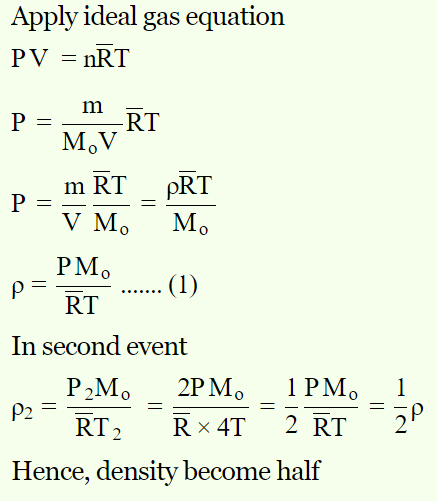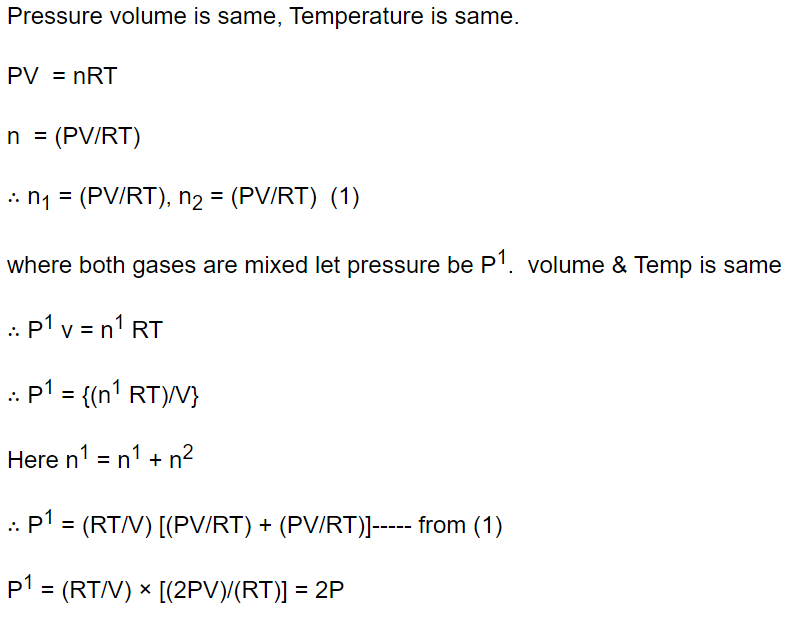## Kinetic Theory Questions and Answers Part-7

1. $O_{2}$ gas is filled in a vessel. If pressure is doubled, temperature becomes four times. How many times its density will become
a) 2
b) 4
c) $\frac{1}{4}$
d) $\frac{1}{2}$

Explanation:2. The volume of a gas will be double of what it is at 0°C (pressure remaining constant) at
a) 1092 K
b) 273 K
c) 546°C
d) 273°C

Explanation: The volume of a gas will be double of what it is at 0°C (pressure remaining constant) at 273°C

3. The pressure and temperature of two different gases is P and T having the volume V for each. They are mixed keeping the same volume and temperature, the pressure of the mixture will be
a) P / 2
b) P
c) 2P
d) 4P

Explanation:4. Three containers of the same volume contain three different gases. The masses of the molecules are $m_{1},m_{2}$   and $m_{3}$  and the number of molecules in their respective containers are $N_{1},N_{2}$   and $N_{3}$  . The gas pressure in the containers are $P_{1},P_{2}$   and $P_{3}$  respectively. All the gases are now mixed and put in one of the containers. The pressure P of mixture will be
a) P < $\left(P_{1}+P_{2}+ P_{3}\right)$
b) $P=\frac{P_{1}+P_{2}+ P_{3}}{3}$
c) $P=P_{1}+P_{2}+ P_{3}$
d) $P>\left(P_{1}+P_{2}+ P_{3}\right)$

Explanation: $P=P_{1}+P_{2}+ P_{3}$

5. At a given volume and temperature, the pressure of a gas
a) Varies inversely as its mass
b) Varies inversely as the square of its mass
c) Varies linearly as its mass
d) Is independent of its mass

Explanation: At a given volume and temperature, the pressure of a gas varies linearly as its mass

6. The rate of diffusion is
a) Faster in solids than in liquids and gases
b) Faster in liquids than in solids and gases
c) Equal to solids, liquids and gases
d) Faster in gases than in liquids and solids

Explanation: The rate of diffusion is faster in gases than in liquids and solids

7. A vessel is filled with an ideal gas at a pressure of 10 atmospheres and temperature $27^{\circ} C$  . Half of the mass of the gas is removed from the vessel and temperature of the remaining gas is increased to $87^{\circ} C$  . Then the pressure of the gas in the vessel will be
a) 5 atm
b) 6 atm
c) 7 atm
d) 8 atm

Explanation:8. When a gas in a closed vessel was heated so as to increase its temperature by $5^{\circ} C$ , there occurred an increase of 1% in its pressure. The original temperature of the gas was
a) $500^{\circ} C$
b) $273^{\circ} C$
c) $227^{\circ} C$
d) $50^{\circ} C$

Explanation: When a gas in a closed vessel was heated so as to increase its temperature by $5^{\circ} C$ , there occurred an increase of 1% in its pressure. The original temperature of the gas was $227^{\circ} C$

9. Two thermally insulated vessels 1 and 2 are filled with air at temperatures $\left(T_{1},T_{2}\right)$  , volume $\left(V_{1},V_{2}\right)$  , and pressure $\left(P_{1},P_{2}\right)$   respectively. If the valve joining the two vessels is opened, the temperature inside the vessel at equilibrium will be
a) $T_{1}+T_{2}$
b) $\left(T_{1}+T_{2}\right)\diagup 2$
c) $\frac{T_{1}T_{2}\left(P_{1}V_{1}+P_{2}V_{2}\right)}{P_{1}V_{1}T_{2}+P_{2}V_{2}T_{1}}$
d) $\frac{T_{1}T_{2}\left(P_{1}V_{1}+P_{2}V_{2}\right)}{P_{1}V_{1}T_{1}+P_{2}V_{2}T_{2}}$b) The temperature must be less than $0^{\circ}C$